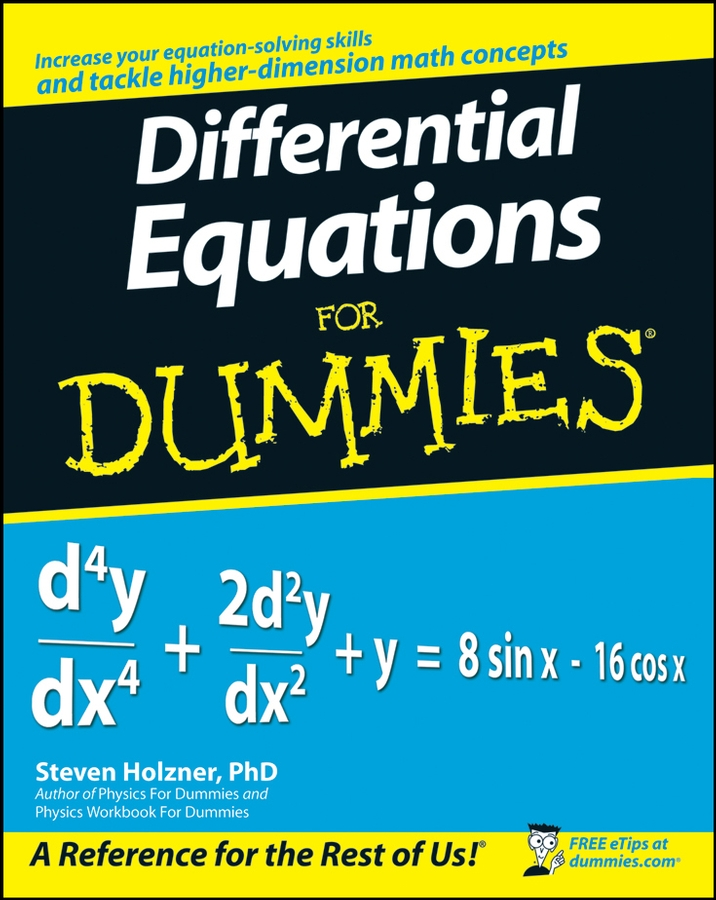# Differential Equations For Dummies

Author:
Steven Holzner
Published: June 3, 2008

## Overview

The fun and easy way to understand and solve complex equations

Many of the fundamental laws of physics, chemistry, biology, and economics can be formulated as differential equations. This plain-English guide explores the many applications of this mathematical tool and shows how differential equations can help us understand the world around us. Differential Equations For Dummies

is the perfect companion for a college differential equations course and is an ideal supplemental resource for other calculus classes as well as science and engineering courses. It offers step-by-step techniques, practical tips, numerous exercises, and clear, concise examples to help readers improve their differential equation-solving skills and boost their test scores.

Differential Equations For Dummies Cheat Sheet

To confidently solve differential equations, you need to understand how the equations are classified by order, how to distinguish between linear, separable, and exact equations, and how to identify homogenous and nonhomogeneous differential equations. Learn the method of undetermined coefficients to work out nonhomogeneous differential equations.